Triangles have so many theorems and rules to remember. If the Pythagorean theorem is so fantastic, then why do we have so many other formulas to remember? Have you heard your teacher mention Soh-Coh-Toa and are still wondering why it is so important? Yeah, you are certainly not alone. The truth is learning the big picture first and then practicing on your own is the best option.

To know what formula to use, we need to break it down into a few simple steps.

1. What are the types of Triangles?

We need to classify a triangle first before we can even attempt to solve for the missing parts.

2. Are you solving for the missing sides or the angles?

Special formulas apply to each case.

3. What are some special cases to remember?

You are going to want to be familiar with special right triangles.

# #1 Let’s Classify the Triangle

Classifying Triangles by Sides

First, a little Latin lesson. Notice the prefix “TRI” meaning three. Three sides and three angles. Perfect! We can now classify the triangles by their sides and angles.  Think of it this way, we usually have a first and last name to set us apart from other people. Triangles will also have a first and last name.

Equilateral- All sides are equal

Isosceles- Two sides are equal

Scalene- No sides are equal

Classifying by Angles

Notice how everything so far has come in “threes”? We can also classify triangles by their angles in three different ways.

Right- Has one 90 degree angle (See special cases)

Acute- Angles are less than 90 degrees

Obtuse- Has an angle that is greater 90 degrees

# #2 Solving for Missing Sides or Angles of a Right Triangle

The Pythagorean Theorem

A right triangle is a special case. There will always be a 90 degree angle so there will be less steps to solve for the missing pieces. Probably not a surprise here that there are three different formulas that you could use. Everything with triangles comes with threes.

Solve for Missing Sides: Use the Pythagorean Theorem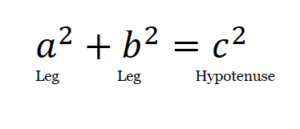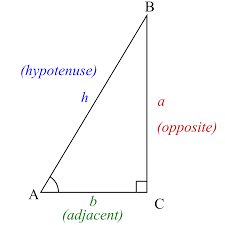# Special Cases of Right Triangles

The Pythagorean theorem will work, but sometimes we love to know extra shortcuts. There are two other ways to solve for missing sides of a right triangle. Again, there are three formulas total to solve for missing sides of right triangles. If you are familiar with them all it will be easier to complete your homework and work more efficiently on a test.

Special case: A 30-60-90 Triangle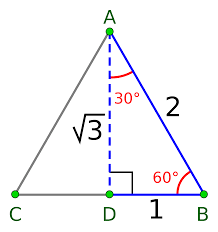In a homework problem, you may be given a right angle and then another angle. If it is 30 or 60 degrees, then you have a 30-60-90 triangle. What does that mean? It means there is a special side ratio that will always apply. So you can use this information to help you.

The 30 degree corresponding side will always be 1

The 30 degree corresponding side will always be square root of 3

The 90 degree corresponding side will always be 2

Special case: A 45-45-90 Triangle

Notice this special right triangle has two equal sides. It could also be referred to as an isosceles right triangle.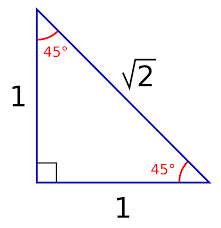You can apply the same idea as discussed in the previous special right triangle. However, the ratios are different with this triangle.

The 45 degree corresponding side will always be 1

The 45 degree corresponding side will always be 1

The 90 degree corresponding side will always be square root of 2

# Solve for Missing Angles of a Right Triangle

Sometimes we know a side, but need to use that information to solve for a missing angle. It might sound challenging, but remember a triangle has three sides and our goal is to find the ratio between each side and angle. In order to do this, we will need three more formulas.

Let’s first look at an example triangle and go over each trigonometric ratio.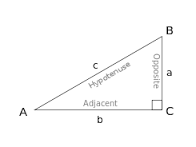Start with a right triangle and find angle A, . The three sides of the triangle are named as follows:

The hypotenuse is the side opposite the right angle, in this case side c. The hypotenuse is always the longest side of a right-angled triangle. It corresponds to angle C.

• The opposite side is the side opposite to the angle we are interested in (angle A), in this case side a.
• The adjacent side is right next to our original angle, but never the hypotenuse. In this case side b.
Now let’s look at the ratios. To remember them, many teachers use SOH-CAH-TOA. Using a trick like this might makes it easier to understand.
Sine = Opposite ÷ Hypotenuse